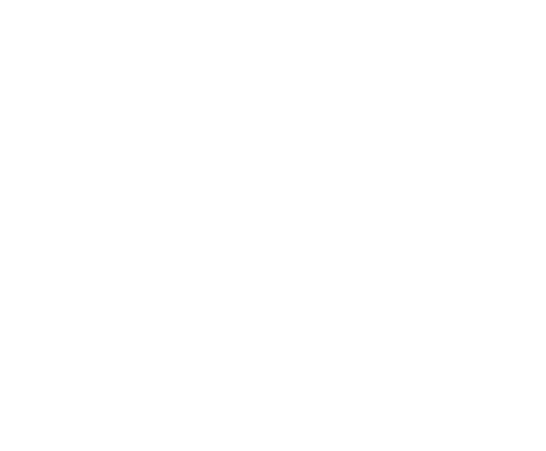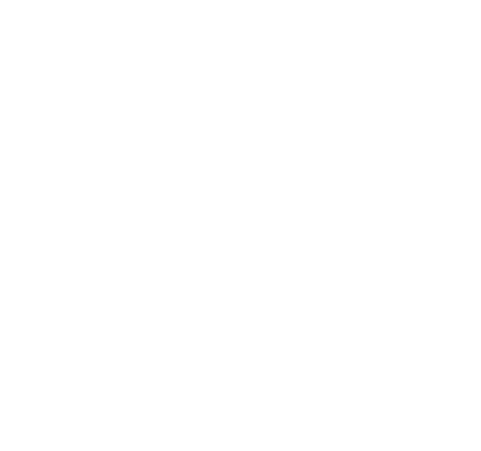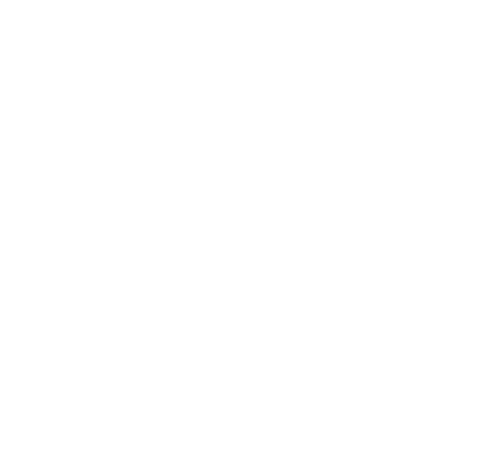Day 10: The Stars Align - Advent of Code 2018

I did the Advent of Code 2018 day 10 challenge in Elixir! Parts one and two are as follows:

``````defmodule Day10 do
def part1(input) do
{grid, _times} =
input
|> parse_input()
|> iter()

draw(grid)
end

def part2(input) do
{_grid, times} =
input
|> parse_input()
|> iter()

times
end

input
|> String.split("\n", trim: true)
end

defp parse_input(input) do
Enum.map(input, fn line ->
[x, y, vx, vy] =
Regex.scan(~r/(-?\d+)/, line, capture: :all_but_first)
|> List.flatten()
|> Enum.map(&String.to_integer/1)

{{x, y}, {vx, vy}}
end)
end

defp iter(grid) do
distance =
grid
|> layout()
|> distance()

iter(grid, distance, 0)
end

defp iter(grid, previous_distance, times) do
new_grid =
Enum.map(grid, fn {{x, y}, {vx, vy} = v} ->
{{x + vx, y + vy}, v}
end)

distance =
new_grid
|> layout()
|> distance()

if distance <= previous_distance do
iter(new_grid, distance, times + 1)
else
{grid, times}
end
end

defp layout(grid) do
Enum.reduce(grid, {0, 0, 0, 0}, fn {{x, _}, _}, {min_x, max_x, min_y, max_y} ->
{
Enum.min([min_x, x]),
Enum.max([max_x, x]),
Enum.min([min_y, x]),
Enum.max([max_y, x])
}
end)
end

defp distance({min_x, max_x, min_y, max_y}) do
abs(max_x - min_x) + abs(max_y - min_y)
end

defp draw(grid) do
grid =
grid
|> Enum.map(&{elem(&1, 0), 0})
|> Enum.into(%{})

{min_x, max_x, min_y, max_y} = layout(grid)

for y <- min_y..max_y do
for x <- min_x..max_x do
if Map.get(grid, {x, y}) do
IO.write("#")
else
IO.write(".")
end
end

IO.write("\n")
end

grid
end
end

# r Day10; :aoc2018 |> :code.priv_dir() |> Path.join("day10.txt") |> Day10.part1()
# r Day10; :aoc2018 |> :code.priv_dir() |> Path.join("day10.txt") |> Day10.part2()``````

Author

Simon Escobar Benitez

Colombian Software Engineer (Erlang Solutions)

Upcoming conferences

Start booking your calendar with more Code Sync conferences happening across the globe. We will be slowly releasing more dates, in the meantime here is what we’ve planned already:

•18 Jul 2019

London

•20 Sep 2019

Budapest

•01 Oct 2019

New York City

•11 Oct 2019

Berlin

•07-08 Nov 2019

London

•29 Nov 2019

Amsterdam

ARTICLES: 8

Day 11: Chronal Charge - Advent of Code 2018I did the Advent of Code 2018 day 11 challenge in Elixir! Parts one and two are as follows:

Day 7: The Sum of Its Parts - Advent of Code 2018I did the Advent of Code 2018 day 7 challenge in Elixir! Parts one and two are as follows:

Day 6: Chronal Coordinates - Advent of Code 2018I did the Advent of Code 2018 day 6 challenge in Elixir! Parts one and two are as follows:

Day 5: Alchemical Reduction - Advent of Code 2018I did the Advent of Code 2018 day 5 challenge in Elixir! Parts one and two are as follows:

Day 4: Repose Record - Advent of Code 2018I did the Advent of Code 2018 day 4 challenge in Elixir! Parts one and two are as follows:

Day 3: No matter how you slice it - Advent of Code 2018I did the Advent of Code 2018 day 3 challenge in Elixir! Parts one and two are as follows:

Day 2: Inventory Management System - Advent of Code 2018I did the Advent of Code 2018 day 2 challenge in Elixir! Parts one and two are as follows: